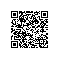# 香农说，要有熵。信息时代由此开启……1948年，香农（Claude Shannon）在贝尔实验室发表论文《通信的数学理论》。文章中提出的数学工具构成了信息论的骨架。香农证明了信源编码的极限是信源的熵，信道编码的极限则是信道容量。

# ◆ ◆ ◆

“二十个问题”游戏# ◆ ◆ ◆# ◆ ◆ ◆

假设有个随机变量 X，它的取值范围 S={1, 2, …, M}，它的概率分布函数是某个定义在S上的函数 P(x)。那么这个随机变量的均值 （更文化点的说法叫数学期望值）就是这样一个东东：

1*P(1)+2*P(2)+3*P(3)+ … +M*P(M).

# ◆ ◆ ◆

很多时候俺们关心的不止一个随机变量，而是很多随机变量。比如，俺们同时关心两个随机变量 X 和 Y，X 的取值范围是 {1, 2}, Y 的取值范围是 {1, 2, 3}。那么俺们可以把这两个随机变量看作一个随机变量对，写作 （X, Y）, 而把它的取值范围理解为所有可能的（X，Y）取值的组合，也就是 {(1, 1), (1, 2), (1, 3), (2, 1), (2, 2), (2, 3)}。把这个集合叫作S，那么这对随机变量就是通过一个定义在S上的概率分布函数 P(x, y) 来描述的。 当这个随机变量对的分布满足 P(x, y)=P(x)P(y) 的时候，俺们就称这两个随机变量是相互独立的。

P(0, 0) = P(0)P(0) = (2/3)(2/3)=4/9

P(0, 1) = P(0)P(1) = (2/3)(1/3)=2/9

P(1, 0) = P(1)P(0) = (1/3)(2/3)=2/9

P(1, 1) = P(1)P(1) = (1/3)(1/3)=1/9

(1/3)(1/3)(2/3)(1/3)(2/3)(1/3)(1/3)(1/3)(1/3)(2/3)=(1/3)^7 * (2/3)^3# ◆ ◆ ◆

“二十个问题”游戏的准确规则及特例

用概率论武装一下之后，同学们应该已经认识到，在“二十个问题”游戏中俺心里想的神秘数字其实就是一个随机变量 X。我们可以假设它的取值范围S={1, 2,…, M} 和概率分布函数 P(x)都 已知。当然在实际情况下我们未必真知道 P(x)，但往往可以大致估计这个函数。如果对这个分布函数我们一无所知，我们不妨认为 P(x) 是个均匀分布。

L(1)=1, L(2)=2, L(3)=3, L(4)=4，

L(5)=5, L(6)=6, L(7)=7, L(8)=7。

（对，L(8)=7，俺没敲错。）

L(1)*P(1)+L(2)*P(2)+…+L(M)*P(M).

# ◆ ◆ ◆

“二十个问题”游戏攒着玩和数据压缩

1. 只要解码端接收到的平均比特数不到这个数字（平均到每个随机变量上），不论用什么编码／解码方法都一定无法重建信息源里蹦出的随机序列。

2. 只要解码端接收到的平均比特数超过这个数字，就一定有一种编码／解码方法可以使解码端重建这个序列。

# ◆ ◆ ◆

1/((1/3)^(n/3)*(2/3)^(2n/3)) = (1/3)^(-n/3)*(2/3)(-2n/3).

- (1/3) log(1/3)- (2/3) log (2/3)。

-P(1)log P(1) - P(2) log P(2) - … - P(M)log P(M).

# ◆ ◆ ◆# ◆ ◆ ◆

“香农的现代信息论永不过时”

20世纪60年代末开始了通信与计算机相结合，通信网迅速发展，人类开始向信息化社会迈进。这就要求信息作业的标准化，加密算法当然也不能例外。标准化对于技术发展、降低成本、推广使用有重要意义。

1949年，香农公开发表《保密系统的通信理论》一文，开辟了用信息论来研究密码学的新思路。这篇文章基于的理论是香农在1945年为贝尔实验室所完成的一篇报告《A Mathematical Theory of Cryptography》。论文发表后，香农被美国政府聘为政府密码事务顾问。

DES

DES全称为Data Encryption Standard，即数据加密标准，是一种使用密钥加密的算法。DES设计中使用的两个分组密码设计原则：混淆（confusion）和扩散(diffusion)，其目的是抗击敌手对密码系统的统计分析。这就很好地提现了香农1949年的论文中所提出的设计强密码思想：

Shannon曾用揉面团来形象地比喻“扩散”和“混淆”的作用，密码算法设计中要巧妙地运用这两个概念。与揉面团不同的是，首先密码变换必须是可逆的，但并非任何“混淆”都是可逆的；二是密码变换和逆变换应当简单易于实现。分组密码的多次迭代就是一种前述的“乘积”组合，它有助于快速实现“扩散”和“混淆”。

“好密码的设计问题，本质上是寻求一个困难问题的解，相对于某种其它条件，我们可以构造密码，使其在过程中的某点上等价于解某个已知数学难题。”

C=W×Log2（1+S/N）使用钉钉扫一扫加入圈子
+ 订阅